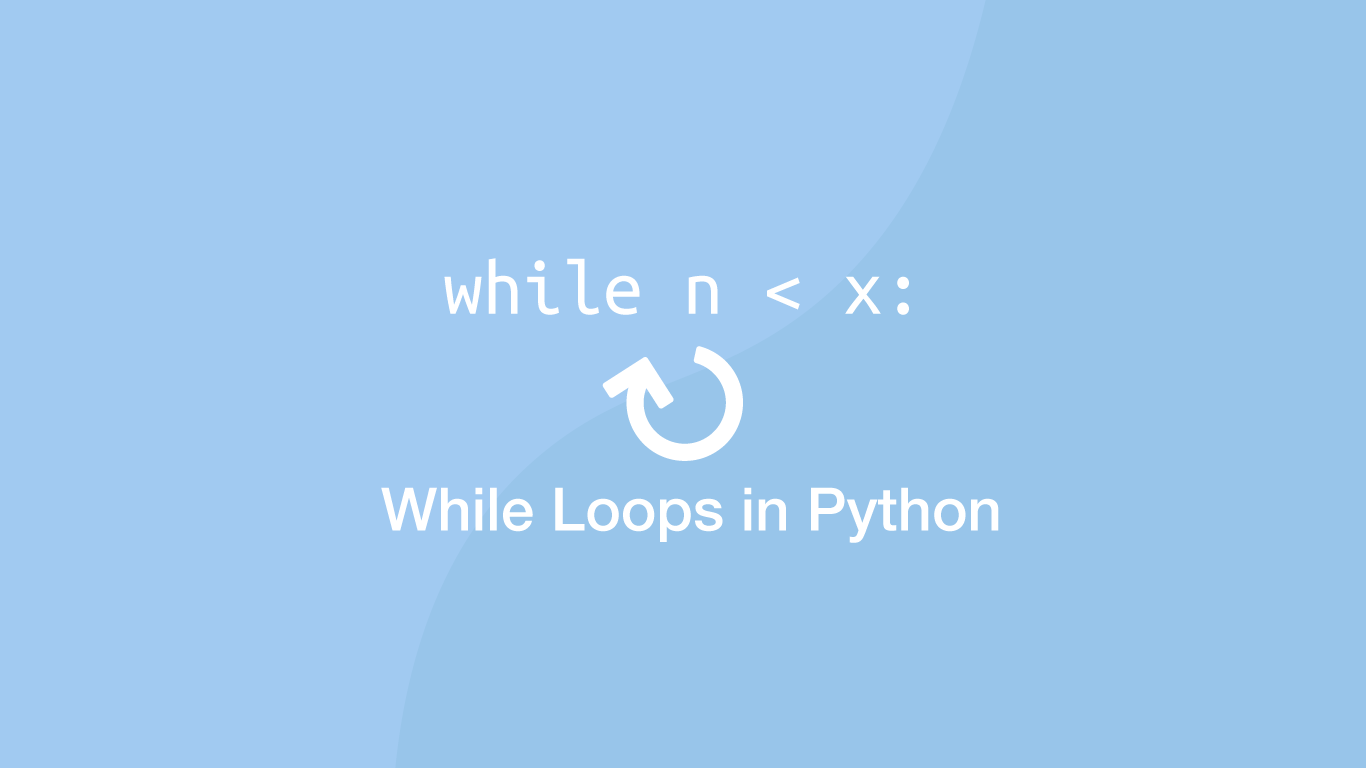# How to Use While Loops in PythonA `while` loop is a looping comparison function available in Python and many other procedural programming languages. We can use them to perform a repetitive task until a condition becomes true. `while` loops are often used instead of for loops when we don't know how many loops are needed beforehand.

In this tutorial, we will learn how to use `while` loops in Python with practical examples.

## The While Loop Syntax

A `while` loop has three main components, an initiator, an expression to evaluate, and indented code that runs inside the loop.

``````while expression:
#do something
``````

## Count Using a While Loop

To begin let's create a `while` loop that will count from `1` to `5` and then finish. Inside the `while` loop, we will print `n` then add `1` to the value `n` on each iteration and finally, print the total.

``````n = 1

while n < 5:
print(n)
n += 1

print('total is: ', 5)
``````
``````1
2
3
4
total is:  5
``````

In the above example, we are setting the variable `n` to `1` and then evaluating that `n` is less than `5` at the start of the `while` loop.

This is true so the code inside `while` runs. Inside the function, we are printing the value of `n` and adding `1` to the value of `n` before looping back to the start of the `while` function and checking if `n` is less than `5`. When `n` is not less than `5` the `while` loop finishes.

## Infinite While Loops

The main thing to watch out for when using `while` loops is that at some point the `while` expression becomes true. If your logic can never be evaluated as true a `while` loop will continue to run until your program crashes. The comparison in the example below can never be true as `n` starts on `0`, is subtracted by `1` inside each `while` loop so `n` will never be greater than `5`.

``````n = 0

while n < 5:
n -= 1

print(n)
``````
``````# There won't be one becuase the program has crashed.
``````

## Break (stop) a While Loop

In light of the potential for infinite loops, we might want to add a “safety net” under certain circumstances. This can be done using a break statement inside some `if` logic.

``````n = 0

while n < 5:
n -= 1
if n == -50:
break

print(n)
``````

In the example above if `n` is equal to `-50` the while loop will finish.

## Loop Though a List Index while a Value is Less than

We can use `while` to loop through a `list` that we know the range of elements it contains but not how many.

Let's say we wanted to count the total of all numbers in a `list` ordered from lowest to highest that are less than a certain value. We could use a function like this:

``````numbers = [2, 4, 6, 8, 10, 12]
n = 0
total = 0

while numbers[n] < 8:
total += numbers[n]
n = n + 1

print(total)
``````
``````12
``````

In the example above, we added the elements together that were less than `8` to reach a total of `12`.

## Multiple Conditions in a while Loop

If a `while` loop runs beyond the number of indexes in a `list` we could add an extra comparison statement using `and`.

``````numbers = [2, 4, 6, 8, 10, 12]
n = 0
total = 0

while n < len(numbers) and numbers[n] < 14:
total += numbers[n]
n = n + 1

print(total)
``````
``````42
``````

## Conclusion

You now know how to use `while` loops in Python, why you might choose them over `for` loops and what to watch out for when using them.

#### Related Tutorials### How to Read a File Line by Line in Python

September 21, 2020### The Python Sleep Function

October 01, 2020### How to use yield Statements in Python

September 28, 2020### How to use while not in Python

April 13, 2021### How to Use Python List Comprehension

October 04, 2020April 07, 2021